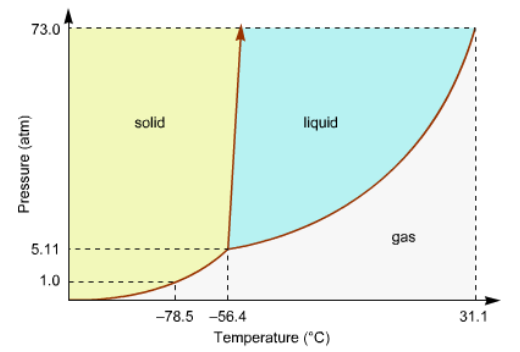# Problem: Consider this phase diagram for carbon dioxide in what phase is CO 2 at 4 atm and -10 °C? a. Solid b. Gas c. Liquid

###### FREE Expert Solution
95% (449 ratings)
###### Problem Details

Consider this phase diagram for carbon dioxide in what phase is CO 2 at 4 atm and -10 °C?

a. Solid

b. Gas

c. LiquidWhat scientific concept do you need to know in order to solve this problem?

Our tutors have indicated that to solve this problem you will need to apply the Phase Diagram concept. You can view video lessons to learn Phase Diagram. Or if you need more Phase Diagram practice, you can also practice Phase Diagram practice problems.

What is the difficulty of this problem?

Our tutors rated the difficulty ofConsider this phase diagram for carbon dioxide in what phase...as low difficulty.

How long does this problem take to solve?

Our expert Chemistry tutor, Dasha took 1 minute and 36 seconds to solve this problem. You can follow their steps in the video explanation above.

What professor is this problem relevant for?

Based on our data, we think this problem is relevant for Professor Hughes' class at UCI.